﻿ 六角冰晶生长过程的相场模拟
«上一篇文章快速检索 高级检索

 哈尔滨工程大学学报2019, Vol. 40Issue (9): 1537-1542  DOI: 10.11990/jheu.2018060670

### 引用本文HAN Duanfeng, WANG Yongkui, JU Lei, et al. Phase field simulation of the hexagonal ice crystal growth process[J]. Journal of Harbin Engineering University, 2019, 40(9): 1537-1542. DOI: 10.11990/jheu.201806067.### 文章历史

Phase field simulation of the hexagonal ice crystal growth process
HAN Duanfeng , WANG Yongkui , JU Lei, WANG Qing , WANG Chunyang
College of Shipbuilding Engineering, Harbin Engineering University, Harbin 150001, China
Abstract: In order to study the microscopic mechanism of ice formation after airborne supercooled water droplets adhere to ship superstructure, numerical simulation of ice crystal growth was carried out in this paper. Based on the classical Kobayashi model in the phase domain method, this paper used the nine-point difference scheme in the finite difference method to discretize the partial differential equation, and realized the simulation and visualization of ice crystal growth with the aid of the Python programming tool. Furthermore, through sensitivity analysis of the main parameters, it was found that some parameters, such as the mean of the interface width and melting temperature, has different influences on ice crystal growth and final morphology. The simulation process of dendrite growth in this paper was consistent with the results of a microscopic experiment, and was similar to the true snowflake profile in nature. This verified the reliability of the numerical method used. Numerical simulation of the ice crystal growth process used in this study will further reveal the micro-mechanism of the ice deposition process by supercooled water droplets.
Keywords: superstructure    icing    droplets    ice crystals    phase field method    finite difference method    numerical simulation    parameter influence

1 Kobayashi相场模型

 $\begin{array}{l} \tau \frac{{\partial P}}{{\partial t}} = - \nabla \left( {{{\left| {\nabla P} \right|}^2}\varepsilon \frac{{\partial \varepsilon }}{{\partial {\mathit{\pmb{n}}}}}} \right) + \nabla ({\varepsilon ^2})\nabla P + \\ \;\;\;\;\;\;\;\;\;\;{\varepsilon ^2}{\nabla ^2}P + {\rm{ }}P\left( {1 - P} \right)\left( {P - 0.5 + m\left( T \right)} \right) \end{array}$ (1)

 $\frac{{\partial T}}{{\partial t}} = {\nabla ^2}T + {\rm{ }}K\frac{{\partial P}}{{\partial t}}$ (2)

2 数值求解过程 2.1 离散形式

 $\frac{{\partial P}}{{\partial t}} = \frac{{{P_{i, j, n + 1}} - {P_{i, j, n}}}}{{\Delta t}} + o(\Delta t)$ (3)

 $\begin{array}{l} {\nabla ^2}P = (2({P_{i + 1, j}} + {P_{i - 1, j}} + {P_{i, j + 1}} + {P_{i, j - 1}}) + \\ \;\;\;\;\;\;\;\;\;\;{P_{i + 1, j + 1}} + {P_{i + 1, j - 1}} + {P_{i - 1, j + 1}} + {P_{i - 1, j - 1}} - \\ \;\;\;\;\;\;\;\;\;\;12{P_{i, j}}){(^2}\Delta {x^2}) - 1 \end{array}$ (4)

2.2 初始条件及边界条件

 ${x^2} + {y^2} \le {r^2}:P = 1, T = 1$ (5)
 ${x^2} + {y^2} > {r^2}:P = 0, T = 0$ (6)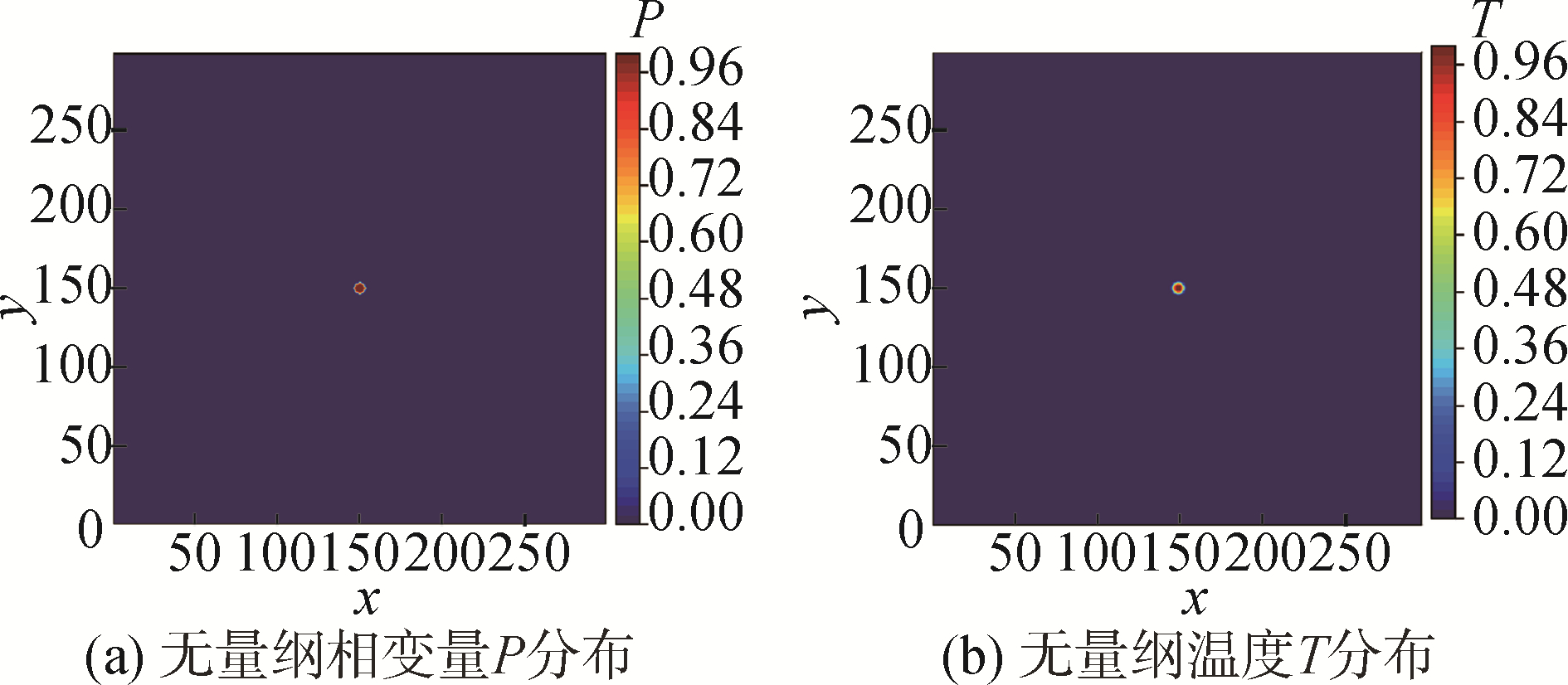Download: 图 1 晶核设置示意 Fig. 1 A schematic diagram of nucleation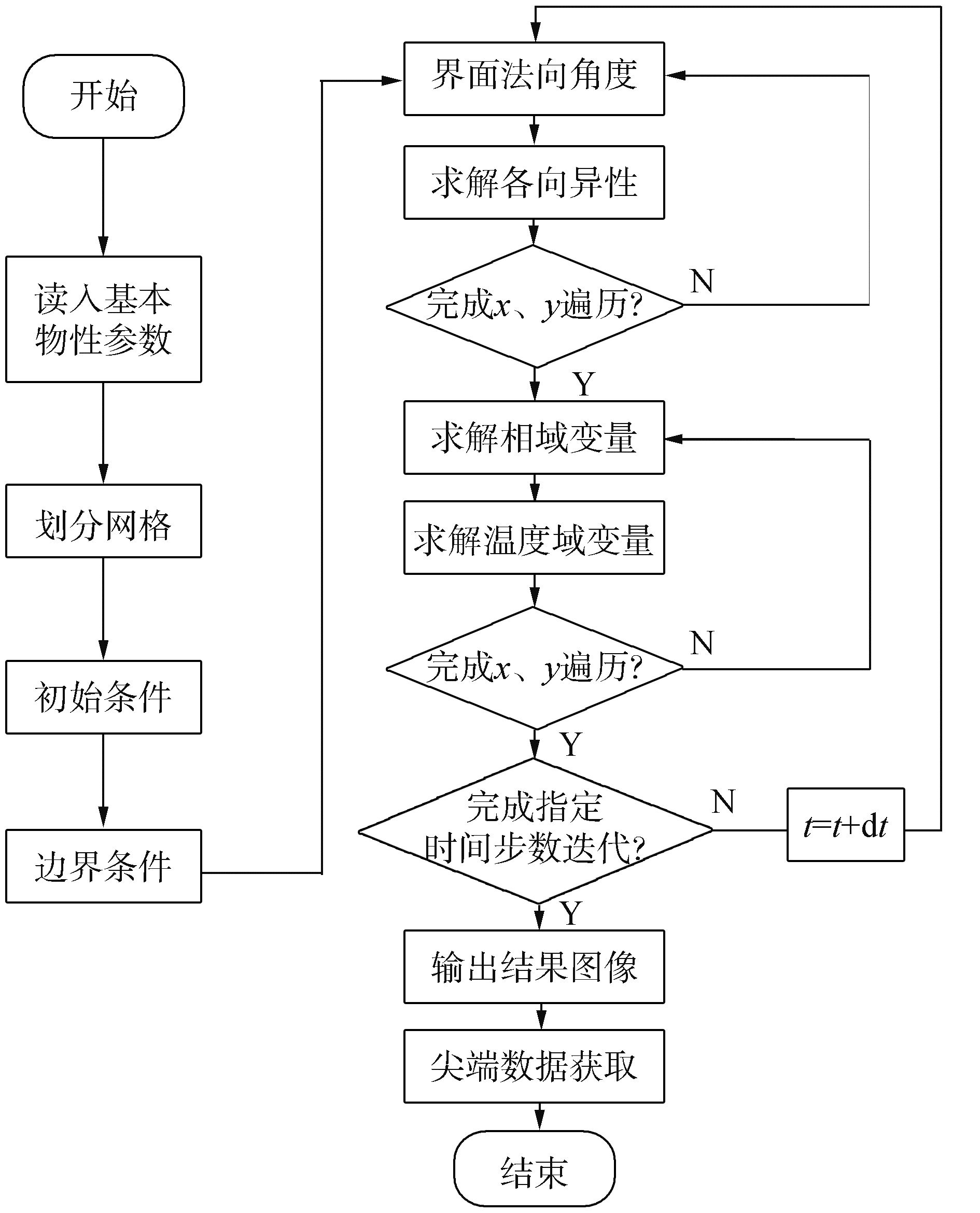Download: 图 2 冰晶计算程序基本流程 Fig. 2 Basic process of ice crystal calculation program
2.3 数值模拟结果及对比 2.3.1 冰晶生长过程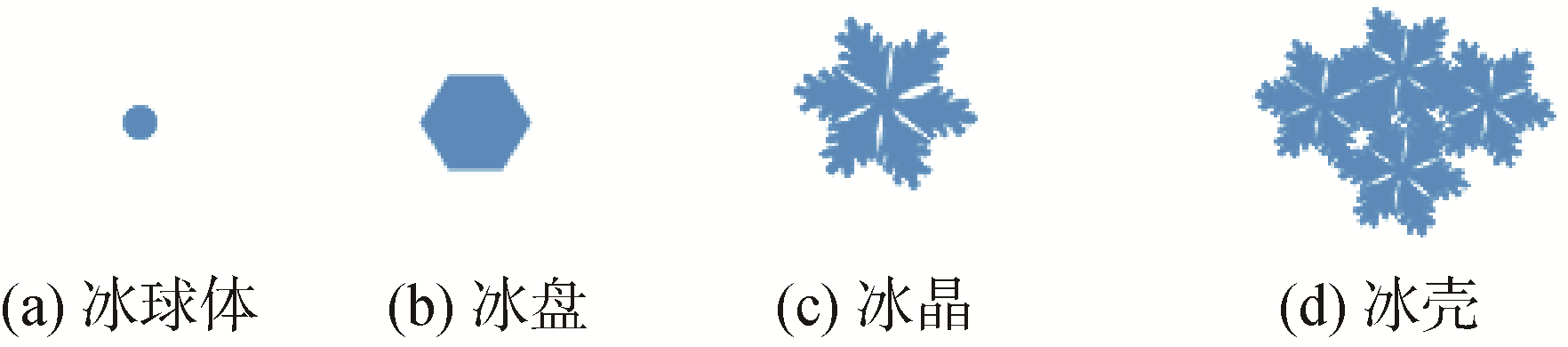Download: 图 3 冰晶生长过程 Fig. 3 The growth process of ice crystals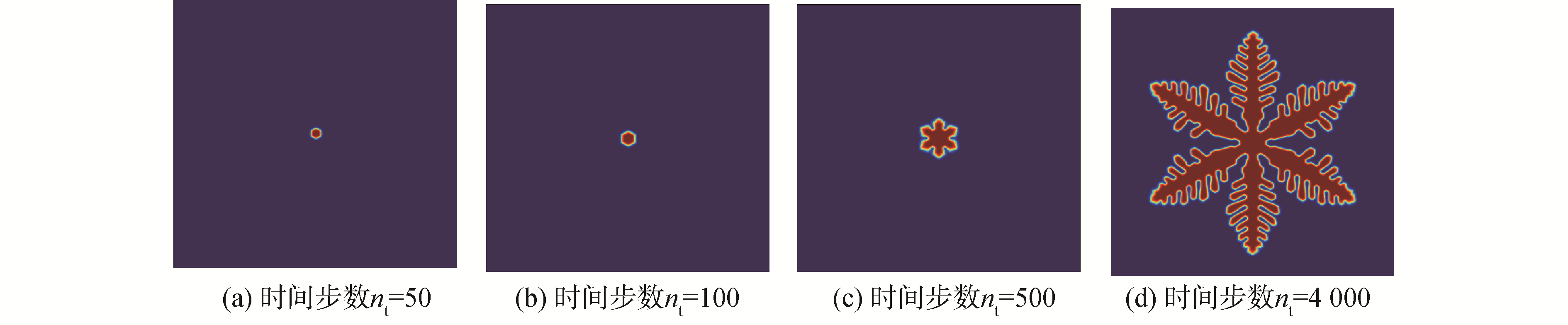Download: 图 4 模拟冰晶生长过程 Fig. 4 A schematic diagram for simulating the growth process of ice crystals
2.3.2 模拟冰晶与自然界雪花对比

Libbrecht展现了其团队拍摄到的形式多样的雪花照片，真实呈现了大自然界中广泛存在的雪花(见图 5(b)), 本文保持上述其他参数不变，划分网格区域为1 000×1 000，迭代1.0 s后获得了如图 5(a)所示的模拟结果，由图 5可见，本文模拟的六角冰晶形貌与大自然中真实存在的雪花是相似的，两图中各主支均生长出或长或短的侧分支，且生长较长的侧分支又生长出新的分支，有效说明了本文相场模型的真实性和准确性。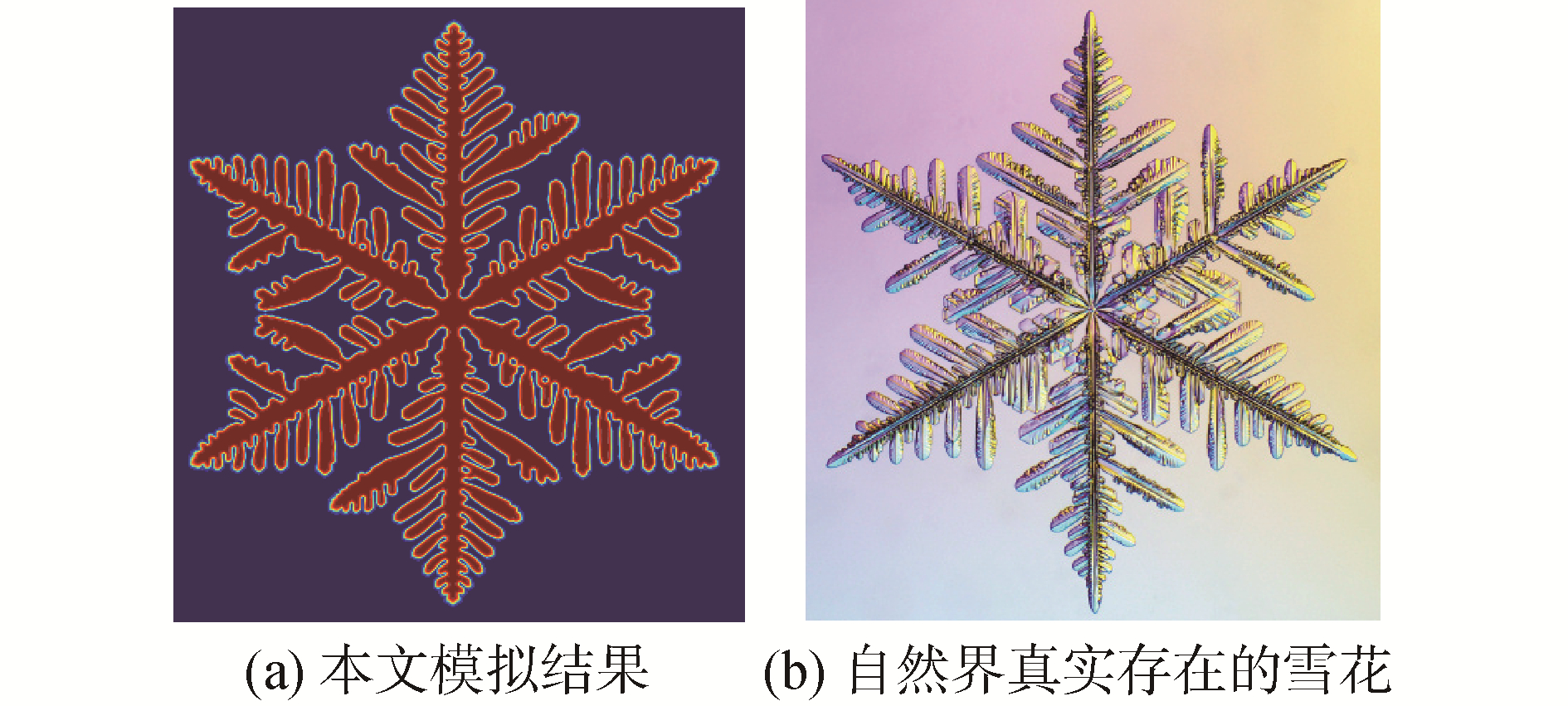Download: 图 5 数值模拟结果与真实雪花对比 Fig. 5 Comparison between numerical simulation results and real snowflakes
3 参数敏感性分析

Kobayashi相场模型中的弛豫时间、各向异性强度、潜热常值等物性参数会影响冰晶生长过程(包括尖端速度、尖端半径等)和冰晶形貌(侧枝大小、取向等)，本文将对模型中各主要参数进行敏感性分析，与真实冰晶形貌进行对比分析，进一步提高冰晶生长模拟的准确性。

3.1 弛豫时间τ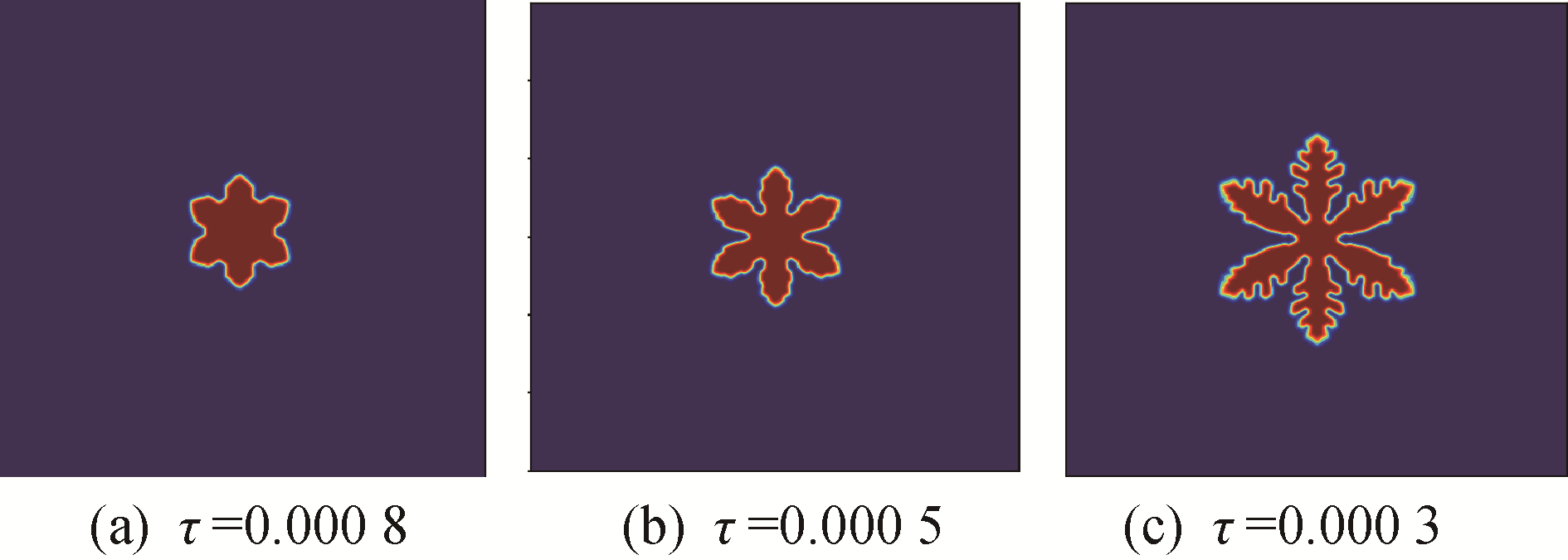Download: 图 6 不同弛豫时间τ模拟结果 Fig. 6 Simulation results of different relaxation time
3.2 界面宽度均值ε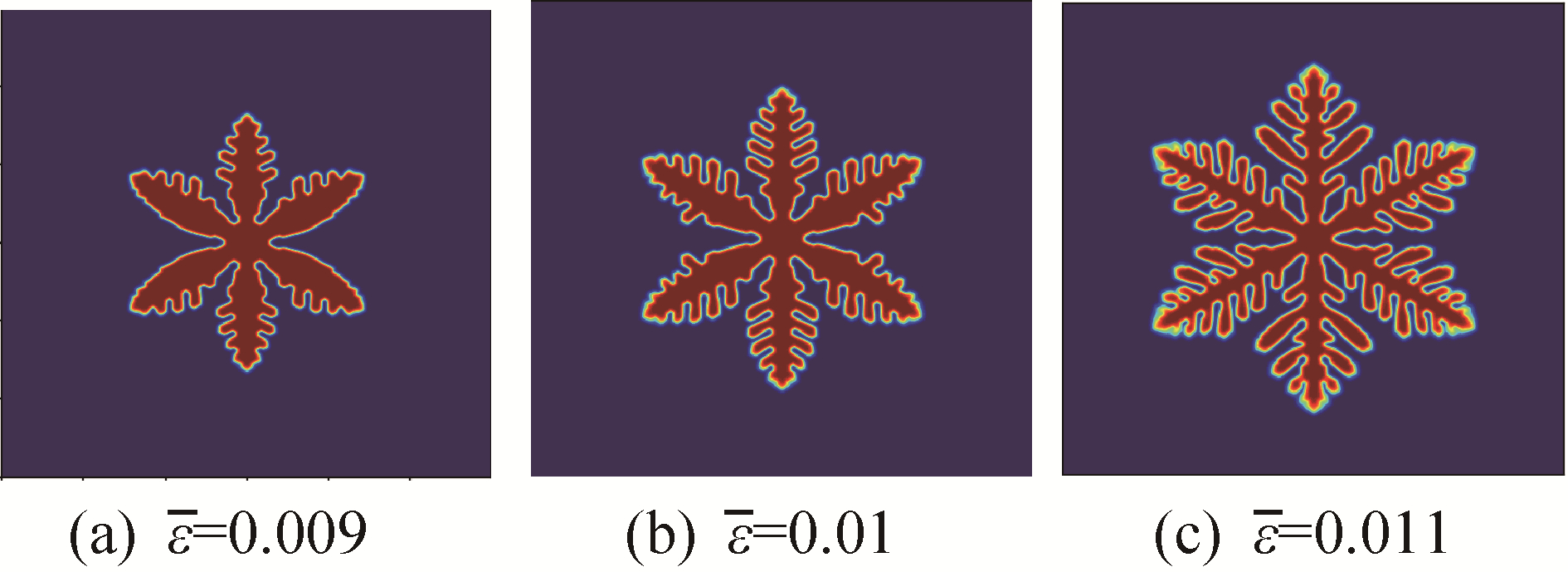Download: 图 7 不同界面宽度均值ε模拟结果 Fig. 7 Simulation results of different mean interface width ε
3.3 融化温度TM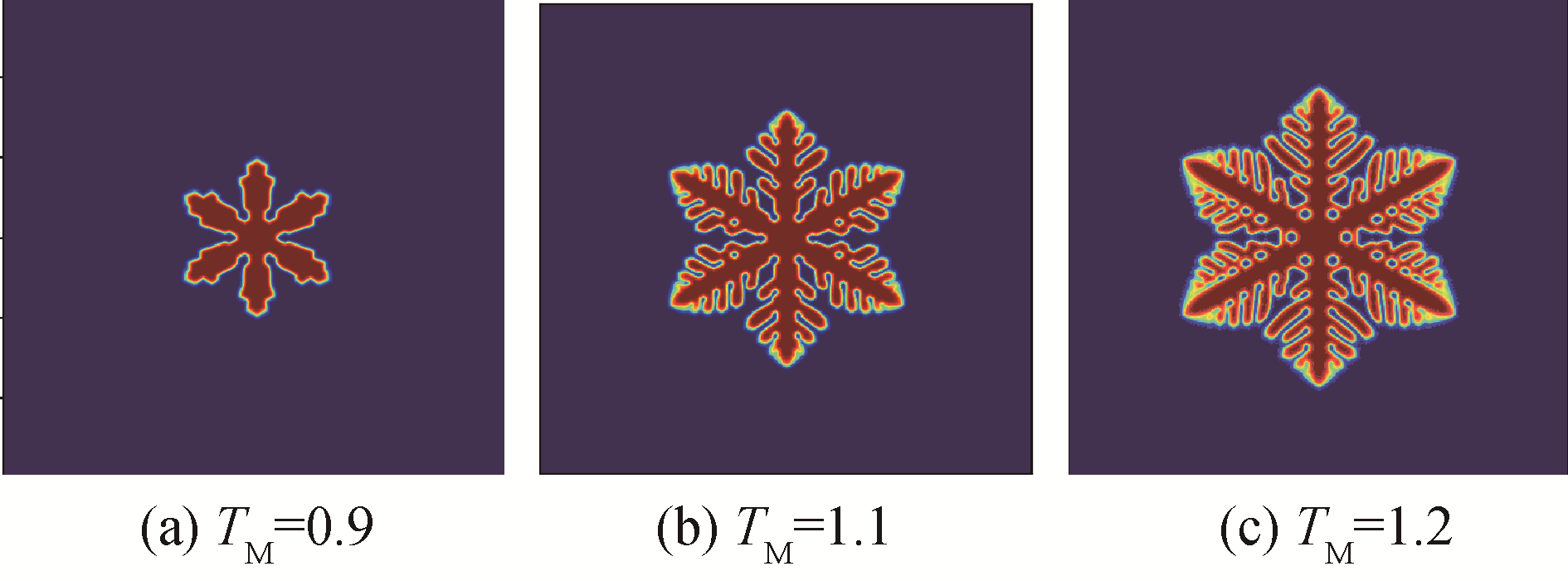Download: 图 8 不同融化温度TM模拟结果 Fig. 8 Simulation results of different melting temperature TM
3.4 各向异性模数j

Kobayashi模型表述界面宽度关于角度的函数时引入了各项异性模数j，其能够影响晶体分支数目及最终形态。本文保持其他参数不变，依次设定j为4、6、8，迭代时间为0.30 s，由图 9可见，模数增加，侧枝的尖端速度及半径也会增加。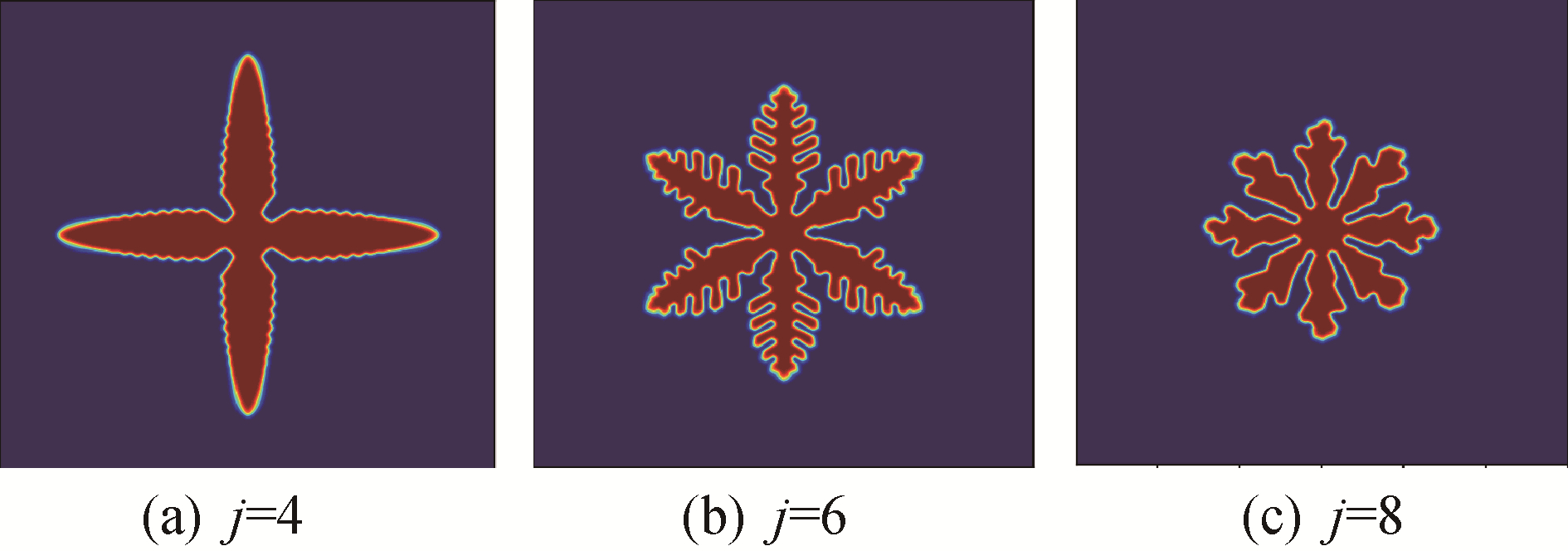Download: 图 9 不同各向异性模数j模拟结果 Fig. 9 Simulation results of different anisotropic modulus j
3.5 各向异性强度δ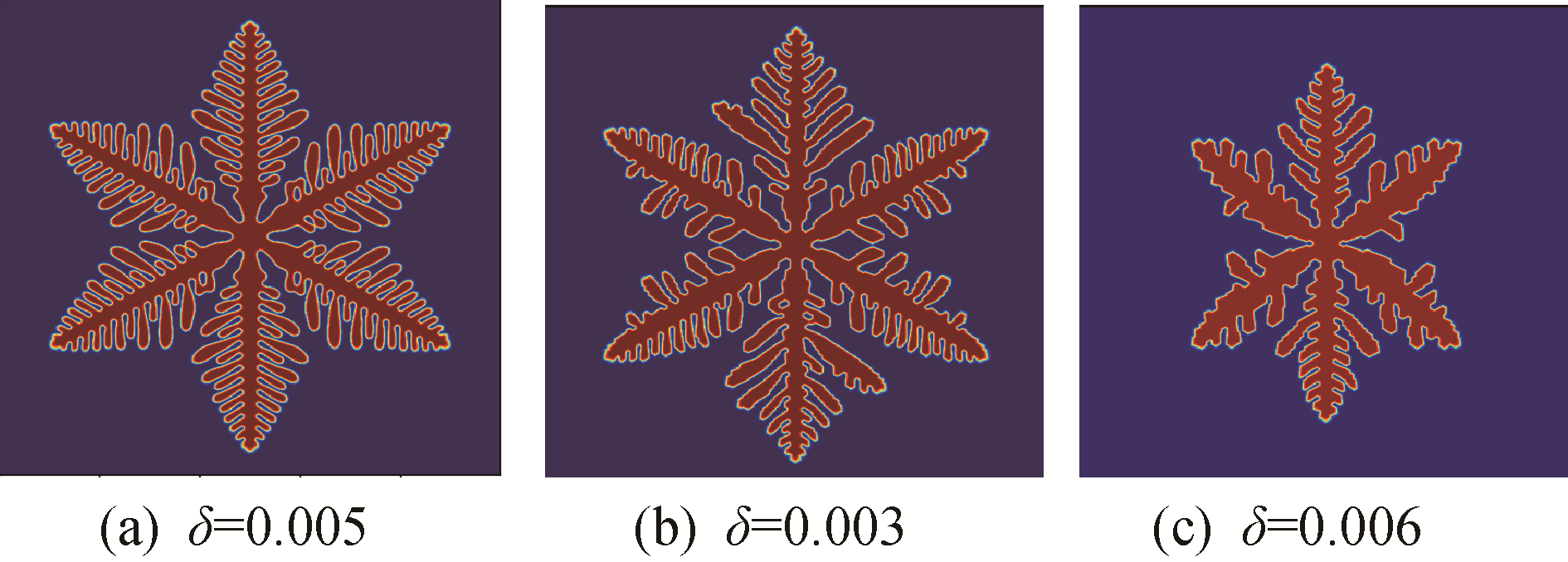Download: 图 10 不同各向异性强度δ模拟结果 Fig. 10 Simulation results of different anisotropic strength δ
3.6 潜热常值KDownload: 图 11 不同潜热常值K模拟结果 Fig. 11 Simulation results of different latent heat constants
4 结论

1) 潜热常值作为影响冰晶生长的最主要参数，其象征着界面扩散层的热量释放，值越大，则能量释放越多，六角冰晶生长越明显，弛豫时间越小则冰晶生长越快，各向异性模数决定了冰晶生长的分支数目，广义上印证了凝固材料杂质的存在，各向异性强度影响冰晶侧分支生长，界面宽度均值则影响着冰晶生长尖端速度及半径。

2) 本文采用的相场模型不仅可以通过耦合相场与外场方程将微观与宏观结合起来，还能模拟分析晶体生长过程中的物性参数(如界面宽度、各向异性强度等)对冰晶生长的影响。

  白响恩, 王建忠, 肖英杰. 中国船舶首次穿越北极东北航道纪实[J]. 航海技术, 2013(1): 2-5. BAI Xiangen, WANG Jianzhong, XIAO Yingjie. Record of Chinese ships crossing the Arctic northeast passage for the first time[J]. Marine technology, 2013(1): 2-5. DOI:10.3969/j.issn.1006-1738.2013.01.001 (0)  丁德文. 工程海冰学概论[M]. 北京: 海洋出版社, 1999: 15-17. DING Dewen. An overview of engineering sea ice[M]. Beijing: China Ocean Press, 1999: 15-17. (0)  KOBAYASHI R. Modeling and numerical simulations of dendritic crystal growth[J]. Physica D: nonlinear phenomena, 1993, 63(3/4): 410-423. (0)  WHEELER A A, BOETTINGER W J, MCFADDEN G B. Phase-field model for isothermal phase transitions in binary alloys[J]. Physical review A, 1992, 45(10): 7424-7439. DOI:10.1103/PhysRevA.45.7424 (0)  WHEELER A A, MURRAY B T, SCHAEFER R J. Computation of dendrites using a phase field model[J]. Physica D: nonlinear phenomena, 1993, 66(1/2): 243-262. (0)  KIM S G, KIM W T, SUZUKI T. Phase-field model for binary alloys[J]. Physical review E, 1999, 60(6): 7186-7197. DOI:10.1103/PhysRevE.60.7186 (0)  QIN R S, WALLACH E R, THOMSON R C. A phase-field model for the solidification of multicomponent and multiphase alloys[J]. Journal of crystal growth, 2005, 279(1/2): 163-169. (0)  陈梅英, 欧忠辉, 卓艳云, 等. 相场法模拟冰晶生长的参数优化[J]. 南京大学学报(自然科学), 2014, 50(6): 873-882. CHEN Meiying, OU Zhonghui, ZHUO Yanyun, et al. Optimization of correlative parameters in numerical simulation of ice crystal growth by phase-field[J]. Journal of Nanjing University (natural science), 2014, 50(6): 873-882. (0)  陈梅英, 陈永雪, 王文成, 等. 冷冻浓缩过程冰晶生长的相场法模拟[J]. 福建农林大学学报(自然科学版), 2010, 39(5): 548-551. CHEN Meiying, CHEN Yongxue, WANG Wencheng, et al. Phase-field simulation of the growth mechanism of ice crystals in the process of freeze concentration[J]. Journal of Fujian Agriculture and Forestry University (natural science edition), 2010, 39(5): 548-551. (0)  陈梅英, 冯力, 欧忠辉, 等. 基于相场法的液态食品冷冻浓缩冰晶生长数值模拟[J]. 农业工程学报, 2014, 30(3): 231-237. CHEN Meiying, FENG Li, OU Zhonghui, et al. Numerical simulation of ice crystal growth of liquid food freeze concentration based on phase-field method[J]. Transactions of the Chinese society of agricultural engineering, 2014, 30(3): 231-237. (0)  卓艳云, 陈梅英, 陈锦权, 等. 各向异性系数对等温结晶冰晶生长相场法模拟的影响[J]. 低温物理学报, 2014, 36(3): 232-237. ZHUO Yanyun, CHEN Meiying, CHEN Jinquan, et al. Influence of anisotropy degree on isothermal crystallization process using phase-field[J]. Chinese journal of low temperature physics, 2014, 36(3): 232-237. (0)  李方方, 刘静, 乐凯. 细胞尺度冰晶生长行为的相场数值模拟[J]. 低温物理学报, 2008, 30(2): 172-175. LI Fangfang, LIU Jing, YUE Kai. Numerical simulation on lce crystal formulation in cellular level based on phase field theory[J]. Chinese journal of low temperature physics, 2008, 30(2): 172-175. (0)  徐立, 陈尚海, 江焕宝, 等. 极地船海水系统冰晶生长对换热器性能影响的相场模拟[J]. 中国修船, 2017, 30(2): 45-48. XU Li, CHEN Shanghai, JIANG Huanbao, et al. The phase field simulation of the influence of heat exchanger performance on ice crystals in sea water piping system of polar ship[J]. China shiprepair, 2017, 30(2): 45-48. (0)  邹阳, 钱华, 梁文清. 制冷剂中冰晶生长的数值模拟[J]. 制冷技术, 2017, 37(2): 64-69. ZOU Yang, QIAN Hua, LIANG Wenqing. Numerical simulation of ice crystal growth in refrigerant[J]. Chinese journal of refrigeration technology, 2017, 37(2): 64-69. DOI:10.3969/j.issn.2095-4468.2017.02.203 (0)  SHAH A, HAIDER A, SHAH S K. Numerical simulation of two-dimensional dendritic growth using phase-field model[J]. World journal of mechanics, 2014, 4(5): 128-136. DOI:10.4236/wjm.2014.45015 (0)  SANAL R. Numerical simulation of dendritic crystal growth using phase field method and investigating the effects of different physical parameter on the growth of the dendrite[J]. arXiv preprint arXiv: 1412.3197, 2014. (0)  陶乐仁, 华泽钊. 低温保护剂溶液结晶过程的显微实验研究[J]. 工程热物理学报, 2001, 22(4): 481-484. TAO Leren, HUA Zezhao. A microscopic study of the crystallization in Cryoprotectent agents[J]. Journal of engineering thermophysics, 2001, 22(4): 481-484. DOI:10.3321/j.issn:0253-231X.2001.04.025 (0)  LIBBRECHT K G. Field guide to snowflakes[M]. Minnesota, USA: Voyageur Press, 2006: 53-63. (0)  BIBEN T. Phase-field models for free-boundary problems[J]. European journal of physics, 2005, 26(5): S47-S55. DOI:10.1088/0143-0807/26/5/S06 (0)